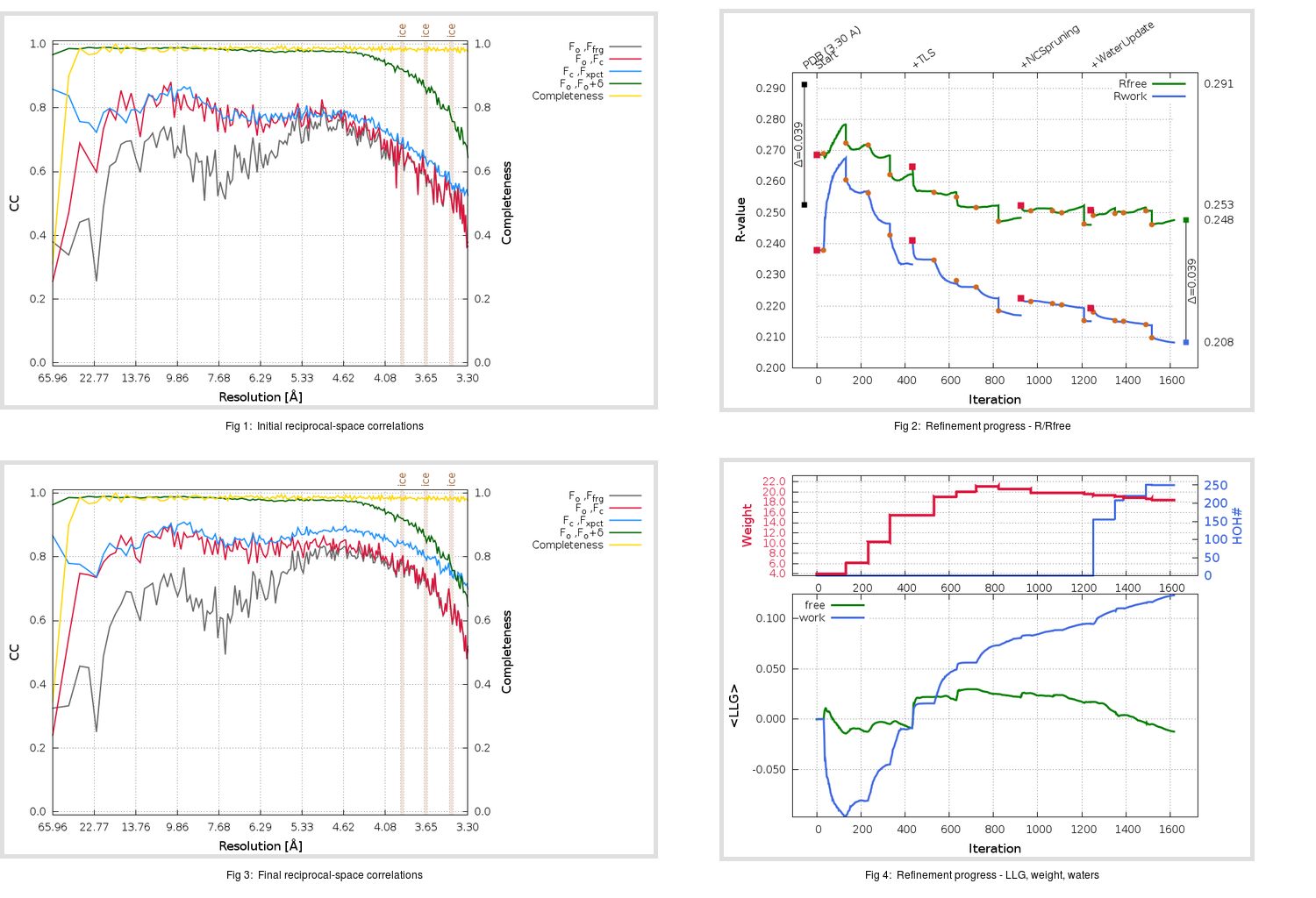Content:

```    Diffraction limits & principal axes of ellipsoid fitted to diffraction cut-off surface:
3.696         0.9263  -0.2496  -0.2824       0.993 a* - 0.118 b* + 0.004 c*
4.200         0.0385   0.8079  -0.5880       0.035 a* + 0.807 b* - 0.589 c*
3.405         0.3749   0.5338   0.7580       0.272 a* + 0.463 b* + 0.844 c*
```

## Deposited

` `
 Date deposited Date data collection Resolution R, Rfree 20151118 3.30 0.2505 0.2913

Molprobity (CCP4 7.0 version) summary:

```Ramachandran outliers =   0.06 %
favored =  96.45 %
Rotamer outliers      =   1.67 %
C-beta deviations     =     0
Clashscore            =   7.23
RMS(bonds)            =   0.0032
RMS(angles)           =   0.87
MolProbity score      =   1.80
Resolution            =   3.30
R-work                =   0.2505
R-free                =   0.2913
```

```Number of waters      =     0

<B> (all atoms) =  142.99 ( sd =   40.86 ) for      27406 non-hydrogen atoms
<B>   (protein) =  143.01 ( sd =   40.87 ) for      27378 non-hydrogen atoms
<B>     (water) =    0.00 ( sd =    0.00 ) for          0 non-hydrogen atoms
<B>    (others) =  127.68 ( sd =   19.17 ) for         28 non-hydrogen atoms

B min/max       (all non-hydrogen atoms) =   37.09 /  454.40
B min/max   (protein non-hydrogen atoms) =   37.09 /  454.40
B min/max     (water non-hydrogen atoms) =    0.00 /    0.00
B min/max     (other non-hydrogen atoms) =   87.13 /  164.16
```

## BUSTER (re-)refinement

` `

Molprobity (CCP4 7.0 version) summary:

```Ramachandran outliers =   1.65 %
favored =  91.07 %
Rotamer outliers      =  11.43 %
C-beta deviations     =     7
Clashscore            =   7.87
RMS(bonds)            =   0.0122
RMS(angles)           =   1.66
MolProbity score      =   2.75
Resolution            =   3.30
R-work                =   0.2080
R-free                =   0.2473
```

```Number of waters      =   250

<B> (all atoms) =   97.29 ( sd =   34.91 ) for      27656 non-hydrogen atoms
<B>   (protein) =   97.82 ( sd =   34.62 ) for      27378 non-hydrogen atoms
<B>     (water) =   39.55 ( sd =   12.86 ) for        250 non-hydrogen atoms
<B>    (others) =   91.24 ( sd =    1.78 ) for         28 non-hydrogen atoms

B min/max       (all non-hydrogen atoms) =   11.24 /  250.16
B min/max   (protein non-hydrogen atoms) =   39.04 /  250.16
B min/max     (water non-hydrogen atoms) =   11.24 /   91.19
B min/max     (other non-hydrogen atoms) =   87.67 /   93.67
```

Refinement progression:Results:

` `
 File Remark 5FO9_aB_refine.01_04_refine.pdb.gz exact refinement commands are in header 5FO9_aB_refine.01_04_refine.mtz.gz including original deposited data and several re-refinement map coefficients 5FO9_aB_refine.01_04_BUSTER_model.cif.gz including any non-standard compound restraints 5FO9_aB_refine.01_04_BUSTER_refln.cif.gz